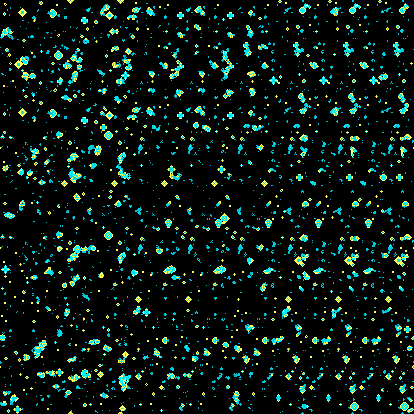# Stateful Animations

The animations discussed so far are stateless, in that each frame could be computed totally independently of any other. For some animations, it may be useful to maintain some state. smoothstep.io allows you to do this using the `iPrevFrame` input variable, which contains the previously rendered frame as a texture.

Enter the following code:

``````int getCell(in ivec2 p) {
// Get the value of the cell in the previous frame at position p. 0 or 1.
ivec2 r = ivec2(textureSize(iPrevFrame, 0));
p = (p + r) % r;
return (texelFetch(iPrevFrame, p, 0 ).x > 0.1 ) ? 1 : 0;
}

float getCellAge(in ivec2 p) {
// Get the y value of the cell, used to simulate the age of the cell, for coloring.
return texelFetch(iPrevFrame, p, 0 ).y;
}

float hash(vec2 p) {
// Computes a psuedo-random number given a vector p.
p = fract(p*vec2(123.34, 456.21));
p += dot(p, p+45.32);
return fract(p.x*p.y);
}

void mainImage(out vec4 fragColor, in vec2 fragCoord) {
ivec2 px = ivec2(fragCoord);
float newCell = 0.;
float age = 0.;

ivec3 c = ivec3(1, -1, 0);

// Conway's Game of Life.
int numNeighbours =   (
getCell(px+c.yy) + getCell(px+c.zy) + getCell(px+c.xy)
+ getCell(px+c.yz)				    + getCell(px+c.xz)
+ getCell(px+c.yx) + getCell(px+c.zx) + getCell(px+c.xx)
);
int thisCell = getCell(px);
newCell = (
((numNeighbours == 2) && (thisCell == 1)) || (numNeighbours == 3)
) ? 1.0 : 0.0;
age = getCellAge(px);
// Decay the age from 1.
age = newCell + 0.97 * (1. - newCell) * age;

// Initialise.
if (iFrame < 2) {
// Set 10% of cells to 1.
newCell = step(0.9, hash(fragCoord));
age = 0.0;
}

float blue = 0.5 - 0.5 * cos(2.0 * newCell + 3.0 * age);
fragColor = vec4(newCell, age, blue, 1.);
}
``````This is an implementation of Conway’s Game of Life. It stores whether a cell is dead or alive using each pixel’s red value - (see `getCell`), and it’s age with its green value (see `getCellAge`).

## The `iFrame` Input Variable

The code runs initialization code in the first few frames, using the condition `if (iFrame < 2) {`. The `iFrame` input variable is an integer, that contains the index of the frame being currently rendered. This is reset to zero at the beginning of the animation, and after every loop (`iTime = 0.0`). If you skip around the animation, its value is not updated. Note that the frame-rate will adapt to the speed of your code/computer, unless you are exporting a video, in which case it is fixed.